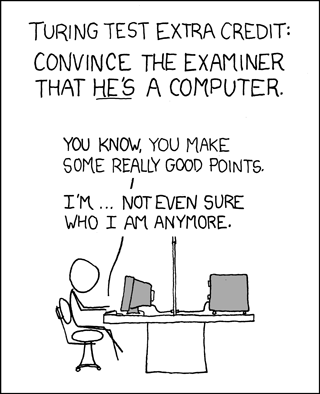Hi All,

As I'm finalizing the CSS conversion of the Math design, I need your help on the images for the 404/Captcha/error pages. You can see the ones I used on the other StackExhange sites.

The idea is to keep it related to the site's topic, but also a bit light-hearted. For example, for Gaming site: Captcha404For Cooking site:

404ErrorYou can append /error, /404, /captcha to see the images from the other newly graduated Stack Exchange sites.

So, what should I use for the Math site? I think it'd be clever to use some in-joke type of math comic strips, assuming they're ok for us to use copy-right wise.

• I think using the "does not exist" symbol ($\not\exists$) for the 404 page would either be really neat or really lame, but I can't tell which. (It would certainly be very hard to make a pretty image out of, which is why I'm not posting this as a serious suggestion.) – Rahul Oct 25 '10 at 3:59
• @Rahul, it doesn't have to be "pretty." The goal is for the users of the site to "get it." An inside joke if you will. I think your suggestion is great. – Jin Oct 25 '10 at 4:01
• For errors, probably the usual suspects e.g. "you divided by zero!"? For the CAPTCHA, display a calculus problem first and then "just kidding, here's the actual CAPTCHA..." – J. M. is a poor mathematician Oct 25 '10 at 6:06
• @J.M. that's pretty clever! can you find a complex calculus formula? maybe i'll put it on blackboard graphic. I think it'd be fitting, since I'm removing the blackboard treatment from the badges. – Jin Oct 25 '10 at 6:20
• I have quite the number of beasts in my stable, and I'll have to look over them to see what's appropriate @Jin. In the meantime, I believe the others have their own monsters they might like to see! (I was thinking of this replacing the "hello fellow robot" image one sees in the CAPTCHA). – J. M. is a poor mathematician Oct 25 '10 at 6:35
• Now I think about something like Venn diagrams. The set of MSE pages and showing that you are out of it. Some kind of figure is better than a symbol, more interesting to view. BTW look at cstheory.stackexchange.com/404 – Yrogirg Jun 8 '12 at 11:01

Following Jin's encouragement, I'm posting my comment as an answer so people can vote on it.

For the 404 page: The "does not exist" symbol. $$\LARGE\unicode{x2204}$$

• Wouldn't $\neg\exists$ be better? – user23211 Jun 8 '12 at 16:53

How about the slogan: "404 is not a very interesting number"

referring to the Ramanujan-Hardy taxi story.

Maybe this XKCD comic for the captcha?Or maybe it's not math-related enough.

Mathematical fallacy for 500 (/error)? For example,

\begin{align} a &= b \\\\ a^2 &= ab \\\\ a^2 - b^2 &= ab - b^2 \\\\ (a+b)(a-b) &= b(a-b) \\\\ a+b &= b \\\\ a+a &= a \quad (∵ a=b) \\\\ 2a &= a \\\\ 2 &= 1\quad?! \end{align}

References:

There might be a rival for $\nexists$, namely the empty set:

$$\LARGE\varnothing$$

Now I wonder if ⊥ was considered for 404 at theoretical computer science.

• I really hope not. – JeffE Jun 10 '12 at 15:59

In the text field below, give a proof that for integers $n \ge 3$, there do not exist integers $x,y,z$ with $x^n + y^n = z^n$.
• Fermat's Last Theorem? shouldn't it be n>=2 ? – Aarthi Jun 8 '12 at 19:01
• @Aarthi Recall the $(3,4,5)$ triangle and other Pythagorean triples. One should also exclude trivial solutions such as $(0,0,0)$ and $(1,0,1)$. – Dylan Moreland Jun 8 '12 at 19:16